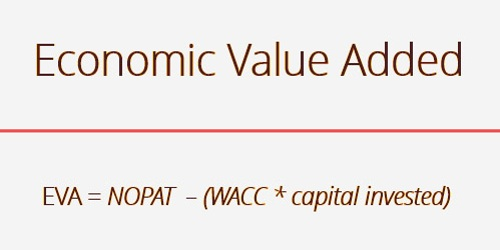FinanceIn corporate finance, Economic Value Added (EVA) or ‘Economic Profit’ is a calculation of the financial performance of a company based on the residual income measured by deducting its capital expenditures from its operating profit, adjusted for cash taxes. The underlying principle is the belief that real productivity happens when additional wealth for shareholders is generated and that ventures will produce returns above their capital costs. EVA is net income minus the capital charge (\$) for raising the capital of the company.

EVA can also be called economic benefit, as it aims to measure a company’s real economic income. The theory is that when the return on the business capital employed by the company exceeds the cost of that capital, value is generated. EVA is the proportional difference in the rate of return over the capital expense of an enterprise. In fact, it is used to calculate the interest a firm produces from the funds invested in it.

EVA adopts almost the same form as residual income and can be expressed as follows:

EVA = NOPAT – (WACC * capital invested)

Where,

NOPAT = Net Operating Profits After Tax

WACC = Weighted Average Cost of Capital

Capital invested = Equity + long-term debt at the beginning of the period

and (WACC * capital invested) is also known as a finance charge

If EVA is negative for a company, it means that the company does not generate value from the funds invested in the business. In contrast, a positive EVA shows a firm produces value from the funds invested in it.

EVA is net operating income after tax (or NOPAT) minus a capital charge, the latter being the sum of the capital expense and economic resources. The basic formula is:

EVA = (ROIC – WACC) . (total assets – current liability)

= (NOPAT – WACC) . (total assets – current liability)

Where:

ROIC= return on invested capital

WACC= weighted average cost of capital

NOPAT= net operating profit after taxes

One primary factor for this element is interest cost adjustment. The cost of interest is included in the finance charge (WACC*capital) that is deducted from NOPAT in the EVA calculation and can be approached in two ways:

Starting with operating profit, then deducting the adjusted tax charges (because tax charge includes the tax break of interest). Therefore, we should always multiply the interest by the charge per unit and add this to the tax charge.

Start with after-tax profit, and return interest net cost. So we should be multiplying the interest charge by (1-tax rate).

EVA calculation:

EVA = net operating profit after taxes – a capital charge (the residual income method)

therefore EVA = NOPAT – (c × capital), or alternatively

EVA = (r × capital) – (c × capital), so that

EVA = (r − c) × capital (the spread method, or excess return method)

Where:

r = rate of return, and

c = cost of capital, or the weighted average cost of capital (WACC).

The equation for EVA shows that there are three key components to a company’s EVA: NOPAT, the quantity of capital invested, and also the WACC. NOPAT is calculated manually but is often listed in a very public company’s financials. Capital invested is the amount of cash accustomed to fund a particular project. WACC is the expected rate of return a company plans to pay its investors; the weights in a company’s capital structure are calculated as a percentage of each financial source. WACC may also be measured but must usually be made available as a public record.

Another definition of EVA can be obtained by looking at the return on net assets (RONA) of a company. RONA could be a ratio that’s calculated by dividing a firm’s NOPAT by the quantity of capital it employs (RONA = NOPAT/Capital) after making the required adjustments of the info reported by a standard financial accounting.

EVA = (RONA – required minimum return) × net investments

If RONA meets the threshold, then EVA is positive. A successful EVA indicates a project produces returns above the minimum return expected. The EVA measure, however, relies heavily on the amount of capital invested and is better used for stable or large, asset-rich firms. Companies with intangible assets, such as technology companies, might not be good candidates for an EVA assessment.

Information Sources: